##1. Pascal's theorem for quadrangles

Consider a quadrangle ABCD inscribed in a conic. Then the two pairs of opposite tangents and the two pairs of opposite sides intersect at four points lying on a line e. The intersection points K, N of the tangents divide harmonically the segment LM defined by the two intersection points of the sides. In addition the pole of line e w.r. to the conic is the intersection point G of the diagonals of the quadrangle.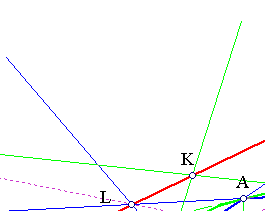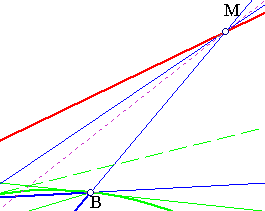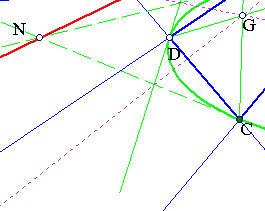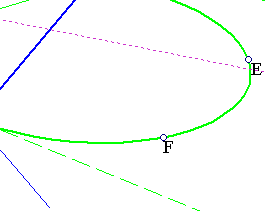The collinearity of the four points K, L, M, N is an immediate consequence of the theorem of Pascal. By the tangency at A, C, G must be on the polar of N. Similarly it is on the polar of K. Thus by the reflective property of polars, K and N must be on the polar of G. The other statement on the harmonicity of the four points (LMKN)=-1 follows from the fact that the polars of M, L are respectively lines LG and MN. Later follows from the basic construction of harmonic points through quadrilaterals (see Harmonic.html ).
See the file CyclicProjective.html for the discussion of the special case of a cyclic quadrangle.

##2. Pascal's theorem for quadrangles, inverse

Consider a quadrangle ABCD and the line L = EF of intersection points {E,F} of pairs of opposite sides. Let {G,H} points of L be harmonic conjugate with respect to {E,F}. Then there is a conic circumscribing the quadrangle and tangent to lines {GB,GD}, {HA,HC} joining points {G,H} with opposite vertices of the quadrangle.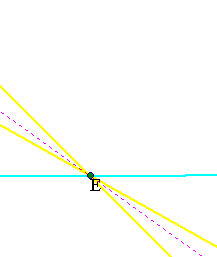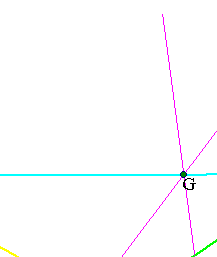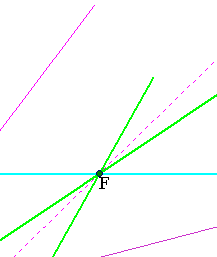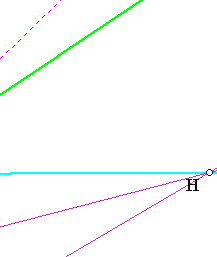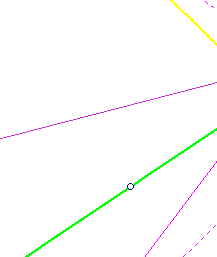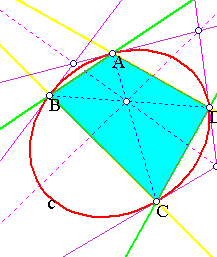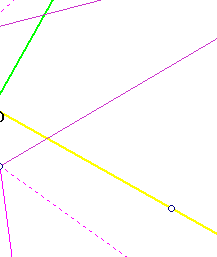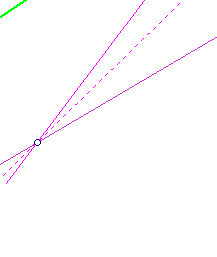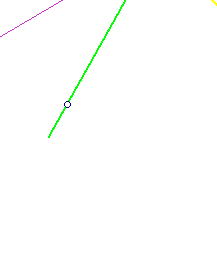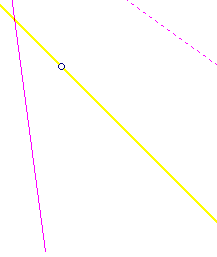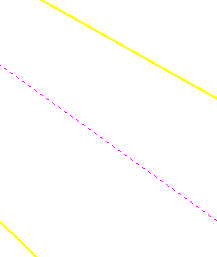Let (c) be the conic passing through {A,B,C,D} and tangent to line AH (see for its existence/construction the file FourPtsAndTangent2.html ). According to the first part the intersection points of line-pairs (AB,CD), (AD,BC) and the tangents at opposite vertices (tB,tD), (tA,tC) are collinear and make a harmonic division, hence coincide with the given four points on L.

CrossRatio.html
CrossRatio0.html
CrossRatioLines.html
CyclicProjective.html
FourPtsAndTangent.html
FourPtsAndTangent2.html
GoodParametrization.html
Harmonic.html
Harmonic_Bundle.html
HomographicRelation.html
Pascal.html
Pascal2.html
PascalOnTriangles.html